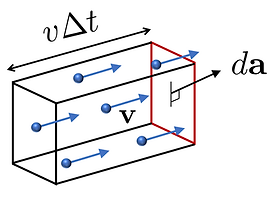## Definition of current density (전류밀도)Consider a swarm of charged particles, each carrying charge qi and moving with velocity vi.

The (volume) current density is defined as## Current through a macroscopic surfaceFor simplicity, let us assume that all charged particles carry the same charge q and move with the same local velocity v. In this case, we havewhere N is the number of particles in volume V and ρ is the volume charge density. The current through the red rectangular frame with infinitesimal area dcan be calculated by considering a close-up view of the region near the frame:The current through surface S is thereforeRemark 1. When the charged particles do not have the same charge or the same velocity, J is still given by the same formula but with an appropriately defined average velocity vavg:and I is still given by Eq. (2).

Remark 2. The definition of J, Eq. (1), describing the current of charges can be generalized to define  volume densities of current for other quantities:

For example, the energy current density per volume iswhere Ei is the energy of the ith particle, and the number current density per volume is